Next: Maclaurin Spheroids Up: Hydrostatics Previous: Rotational Hydrostatics

# Equilibrium of a Rotating Liquid Body

Consider a self-gravitating liquid body in outer space that is rotating uniformly about some fixed axis passing through its center of mass. What is the shape of the body's bounding surface? This famous theoretical problem had its origins in investigations of the figure of a rotating planet, such as the Earth, that were undertaken by Newton, Maclaurin, Jacobi, Meyer, Liouville, Dirichlet, Dedekind, Riemann, and other celebrated scientists, in the 17th, 18th, and 19th centuries (Chandrasekhar 1969). Incidentally, it is reasonable to treat the Earth as a liquid, for the purpose of this calculation, because the shear strength of the solid rock out of which the terrestrial crust is composed is nowhere near sufficient to allow the actual shape of the Earth to deviate significantly from that of a hypothetical liquid Earth (Fitzpatrick 2012).

In a co-rotating reference frame, the shape of a self-gravitating, rotating, liquid planet is determined by a competition between fluid pressure, gravity, and the fictitious centrifugal force. The latter force opposes gravity in the plane perpendicular to the axis of rotation. Of course, in the absence of rotation, the planet would be spherical. Thus, we would expect rotation to cause the planet to expand in the plane perpendicular to the rotation axis, and to contract along the rotation axis (in order to conserve volume).

For the sake of simplicity, we shall restrict our investigation to a rotating planet of uniform density whose outer boundary is ellipsoidal. An ellipsoid is the three-dimensional generalization of an ellipse. Let us adopt the right-handed Cartesian coordinate system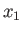,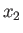,. An ellipse whose principal axes are aligned along the- and-axes satisfies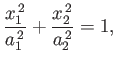(2.94)

whereandare the corresponding principal radii. Moreover, as is easily demonstrated,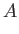(2.95)(2.96)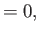(2.97)

whereis the area,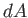an element of, and the integrals are taken over the whole interior of the ellipse. Likewise, an ellipsoid whose principal axes are aligned along the-,-, and-axes satisfies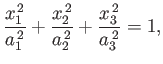(2.98)

where,, and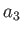are the corresponding principal radii. Moreover, as is easily demonstrated,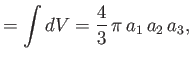(2.99)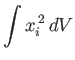(2.100)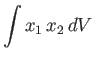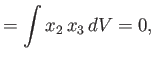(2.101)

whereis the volume,an element of, and the integrals are taken over the whole interior of the ellipsoid.

Suppose that the planet is rotating uniformly about the-axis at the fixed angular velocity. The planet's moment of inertia about this axis is [cf., Equation (2.100)]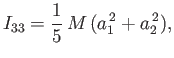(2.102)

where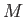is its mass. Thus, the planet's angular momentum is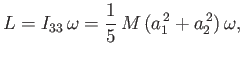(2.103)

and its rotational kinetic energy becomes(2.104)

According to Equations (2.83) and (2.84), the fluid pressure distribution within the planet takes the form(2.105)

where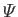is the gravitational potential (i.e., the gravitational potential energy of a unit test mass) due to the planet,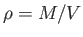the uniform planetary mass density, and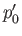a constant. However, it is demonstrated in Appendix D that the gravitational potential inside a homogeneous self-gravitating ellipsoidal body can be written (Chandrasekhar 1969; Lamb 1993)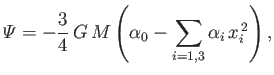(2.106)

where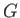is the universal gravitational constant (Yoder 1995), and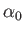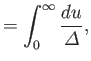(2.107)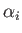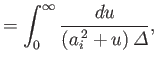(2.108)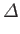(2.109)

Thus, we obtain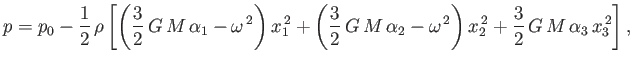(2.110)

where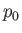is the central fluid pressure. The pressure at the planet's outer boundary must be zero, otherwise there would be a force imbalance across the boundary. In other words, we require(2.111)

whenever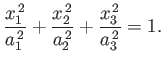(2.112)

The previous two equations can only be simultaneously satisfied if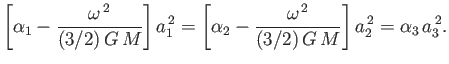(2.113)

Rearranging the previous expression, we obtain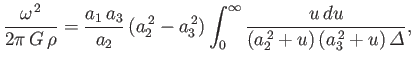(2.114)

subject to the constraint(2.115)

where use has been made of Equation (2.99).

Finally, according to Appendix D, the net gravitational potential energy of the planet is(2.116)

Hence, the body's total mechanical energy becomes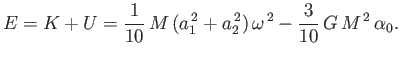(2.117)Next: Maclaurin Spheroids Up: Hydrostatics Previous: Rotational Hydrostatics
Richard Fitzpatrick 2016-03-31